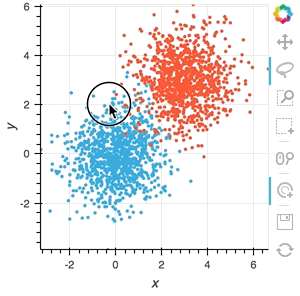# Selection1d paired ¶

Title
Multiple selection streams example
Description
A linked streams example demonstrating how to use multiple Selection1D streams on separate Points objects.
Backends
Bokeh
Tags
streams, linked, selection, interactive
In [ ]:
import numpy as np
import holoviews as hv
from holoviews import opts, streams

hv.extension('bokeh')

In [ ]:
# Declare two sets of points generated from multivariate distribution
points = hv.Points(np.random.multivariate_normal((0, 0), [[1, 0.1], [0.1, 1]], (1000,)))
points2 = hv.Points(np.random.multivariate_normal((3, 3), [[1, 0.1], [0.1, 1]], (1000,)))

# Declare two selection streams and set points and points2 as the source of each
sel1 = streams.Selection1D(source=points)
sel2 = streams.Selection1D(source=points2)

# Declare DynamicMaps to show mean y-value of selection as HLine
hline1 = hv.DynamicMap(lambda index: hv.HLine(points['y'][index].mean() if index else -10), streams=[sel1])
hline2 = hv.DynamicMap(lambda index: hv.HLine(points2['y'][index].mean() if index else -10), streams=[sel2])

# Combine points and dynamic HLines
(points * points2 * hline1 * hline2).opts(
opts.Points(active_tools=['box_select', 'tap'], height=400,
tools=['box_select', 'lasso_select', 'tap'], width=400))